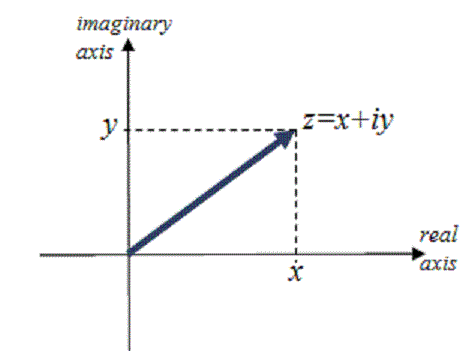# Section 4.3 The Complex Plane

Section Objectives

1. Plot complex numbers in the complex plane.
2. Compute the absolute value of a complex number.
3. Perform operations on complex numbers in the complex plane.

### The Complex Plane

The complex plane is identical to the $(x,y)$-rectangular coordinate plane, except that the $x$-axis is replaced by the real axis, the $y$-axis is replaced by the imaginary axis. The complex number $z=x+yi$ is plotted as the $(x,y)$ ordered pair.The absolute value of the complex number $a+bi$ is the distance in the complex plane from the origin to $a+bi$: $\quad |a+bi| = \sqrt{a^2+b^2}$.

#### Examples

• Let $z=3+4i$. Compute $|z|$.

• Compute $|7-6i|$.

### Arithmetic Operations in the Complex Plane

Most of the operations that can be performed on complex numbers can be illustrated in the complex plane. For example, addition and subtraction can be illustrated with triangles and parallelograms, and conjugation is a reflection about the real axis.

• Let $x=2+3i$ and $y=3-i$. In the complex plane, illustrate $x+y$ and $x-y$.

• Let $z=3+4i$. Show $z$, $\bar{z}$ and $-z$ in the complex plane.

• Let $u=5+i$ and $v=-3+4i$. Find the distance from $u$ to $v$, and find the complex number that is the midpoint of $u$ and $v$.